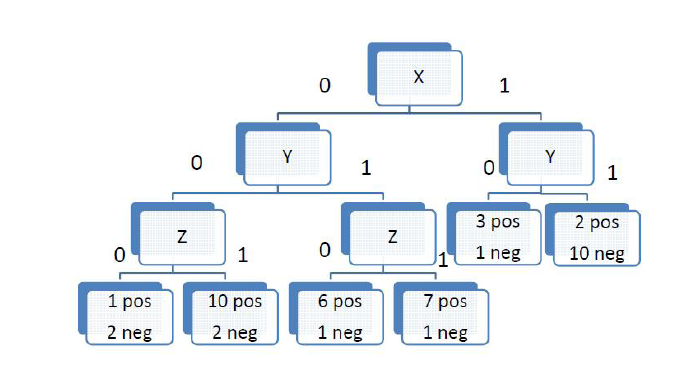Lab 9: Learning Theory and non-parametric learning

Part 0: Learning Theory

1. You are making the classifier for faces, and run your first test, and discover that your test error is 0.42, and training error is 0.35. What does this mean in terms of what the error actually is? What tests can you run to see what the cause of the error is? For each type of problem, what could you do to solve it? Lekan has another set of 100 more labeled photographs of faces. Would that help? Why or why not?
2. Let’s talk about another way to prune decision trees. Let’s say we have a data set with 100 binary attributes. Let there be exactly one example for every possible truth assignment for the 100 binary attributes,$a_1$ to$a_{100}$. This gives us$2^{100}$ training examples. For the response variables, we will just let that be the same as$a_1$ for 99% of the examples.
1. What should a desired decision tree for this data set probably look like?
2. After you theoretically build a complete decision tree (using all the attributes), what will the tree look like?
3. We introduce this pruning strategy: Let’s call the complete tree$T_0$. We then prune (chop off) a subtree (can be leaf or interior node) of that tree that, after it is pruned, results in the lowest apparent increase in the prediction error rate of the whole tree. This tree is called$T_1$. Apparent increase in error,$\alpha = \displaystyle\frac{error_{pruned} - error_{unpruned}}{|nodes_{unpruned}|-|nodes_{pruned}|}$. Repeat, so you obtain$T_0, T_1, T_2, \ldots , T_n$, where$T_n$ corresponds to the “tree” that has no nodes (we just predict by taking the mode). We then estimate the generalization error of each pruned tree, and pick the best one. Yay for holding out a test set!
1. Given the decision tree below, where we are using the attributes X, Y, and Z, find the pruned trees$T_0, T_1, \ldots , T_n$. As an example, if we prune the left-most node corresponding to a decision on Z, we will replace that node with a leaf with 11 positive and 4 negative examples, so if any examples reach that nodes, we will classify positive, since it’s the majority.
2. Say our test set  consists of the following four, in the form (X,Y,Z,value): (1,1,1,0), (1,0,0,0), (0,1,1,1), (0,0,0,1). Which tree has the best generalization error?
3. Kernels!
1. Show an example of four points (with coordinates) in$\Re^2$ that are not linearly separable, but can be separated quadratically.
2. Apply an$\Re^2 \rightarrow \Re$ transform to the y so they are linearly separable. That is, replace every point (x,y) with another point (x, f(x,y)), where the function y is of your choosing.
3. Now note that this is similar to putting these 2D points in 3-space. That is, instead of the points (x,y), we defined a kernel function, K, so we can represent our point in 3-space as$(x, y, K(x,y))$. So, now in 3-space, our point is linearly separable. Using the$K=f(x,y)$ you devised earlier, draw your new points in 3-space.
4. Now, draw 5 or 6 points in 2D that cannot be linearly separated in 2D, and cannot be separated in 3D even after applying a kernel. Show that they are linearly separably in 4D, using two kernels,$K_1$, and$K_2$, and four-dimensional points$(x, y, K_1(x,y), K_2(x,y))$.
5. Hence, we see that we can use multiple kernels to transform our data into higher-dimensional space to be linearly separable. In this way, we can simulate using any type of classifier using just linear classifiers. Also note that in higher-dimensional space, we are prone to the curse of dimensionality, and by using kernels, we still have the same problem of overfitting, just as if we used a high-order polynomial instead of a linear classifier.Part 1: Learning Strategies

1. For each of the following, determine what learning algorithm(s) you would use to classify or cluster the data. If a search or gradient descent is required, decide which one to use. It if helps in clarifying give examples of features, response variables, and other characteristics about your training set.
1. Finding a fingerprint match in an FBI database.
2. Determining whether an object is a table or a chair.
3. Finding cliques at your high school.
2. Give an example of a simple dataset where KNN fails, but a decision tree would classify correctly.
3. One important part of using a KNN classifier is the choice of K. What are the advantages and disadvantages of using a small or large value for k?

Part II: FaceThingy, finished

After your decision tree learning algorithm is complete, the last part is to implement a cascaded AdaBoost.

1. Implement AdaBoost. It will be useful to store a List of weights along with the list of examples.
2. When classifying faces, this may still be slow. So, train a series of decision trees. For the first one, made an accuracy threshold of around 0.6. With subsequent trees, train only on the data that makes it past the tree before it. In this way, you essentially have a series of classifiers a face must go through to be classified a face. This is called a cascade.
3. When you do classify a face,
4. Two extensions
1. There are features other than Haar-like features. Look up some of them and implement them.
2. Face recognition. We are only currently doing face detection. Face recognition can use the same strategy. Simple systems will detect parts of faces–eyes, edges of lips, tip of nose, eyebrows, edge of eyebrows, etc. It then finds the distances between these facial features, and uses those as attributes to differentiate between different faces. Use something like this to recognize different faces.

One response to “Lab 9: Learning Theory and non-parametric learning”

1.I sense a disturbance in the force of parsing in those subscripts and superscripts.